Algebra 1 1-8 Complete Lesson: An Introduction to Equations
starstarstarstarstarstarstarstarstarstar
5 (1 rating)
by Matthew Richardson
| 29 Questions
Note from the author:
A complete formative lesson with embedded slideshow, mini lecture screencasts, checks for understanding, practice items, mixed review, and reflection. I create these assignments to supplement each lesson of Pearson's Common Core Edition Algebra 1, Algebra 2, and Geometry courses. See also mathquest.net and twitter.com/mathquestEDU.The outlined content above was added from outside of Formative.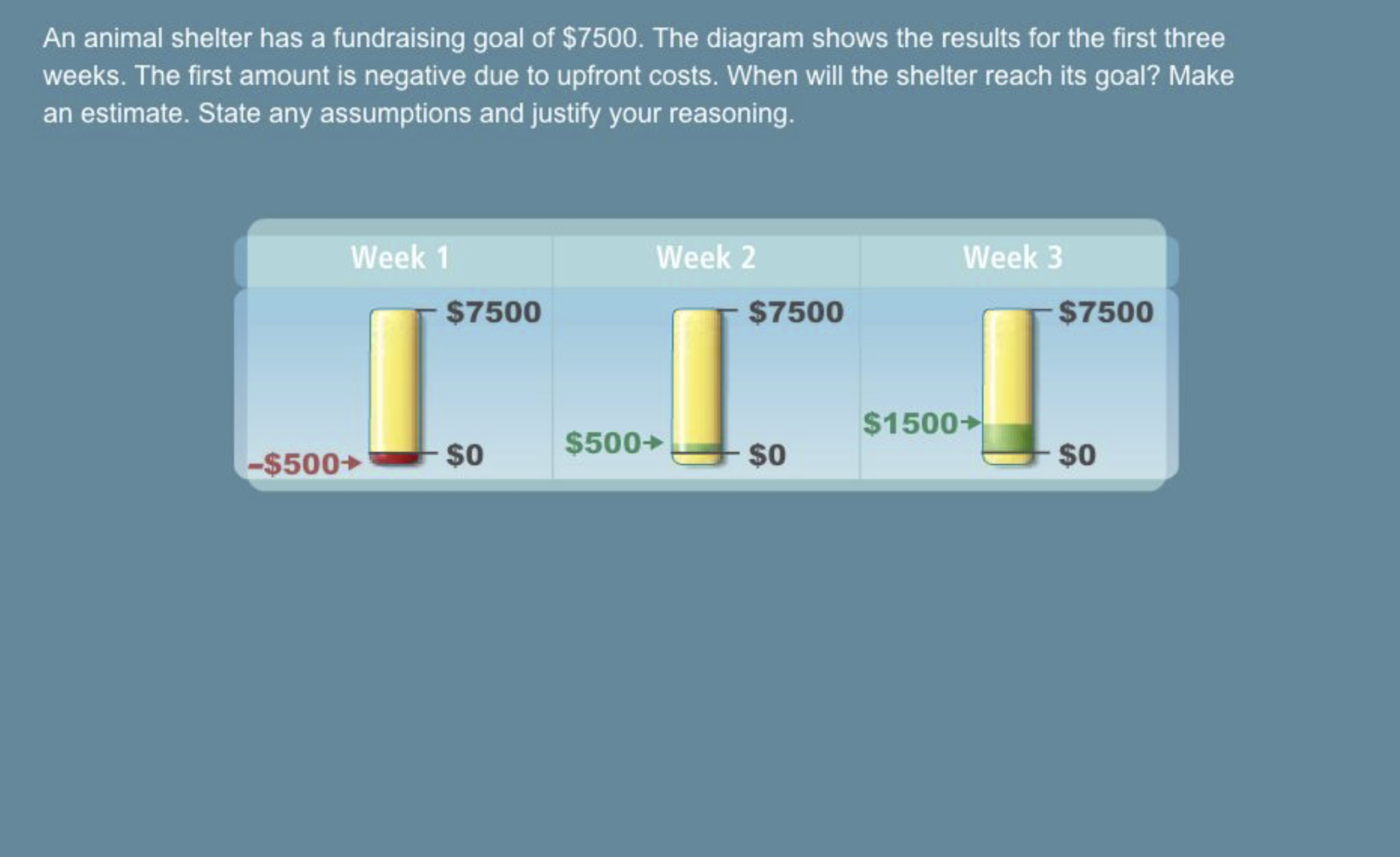1
1
10 pts
Solve It! If this trend continues, during which week will the shelter reach its goal?

Enter only the week number.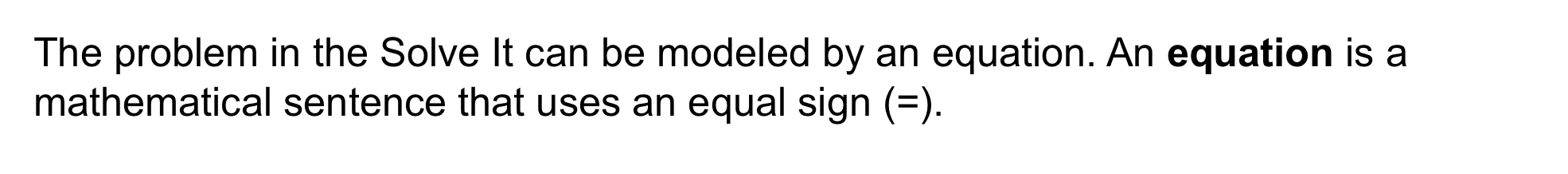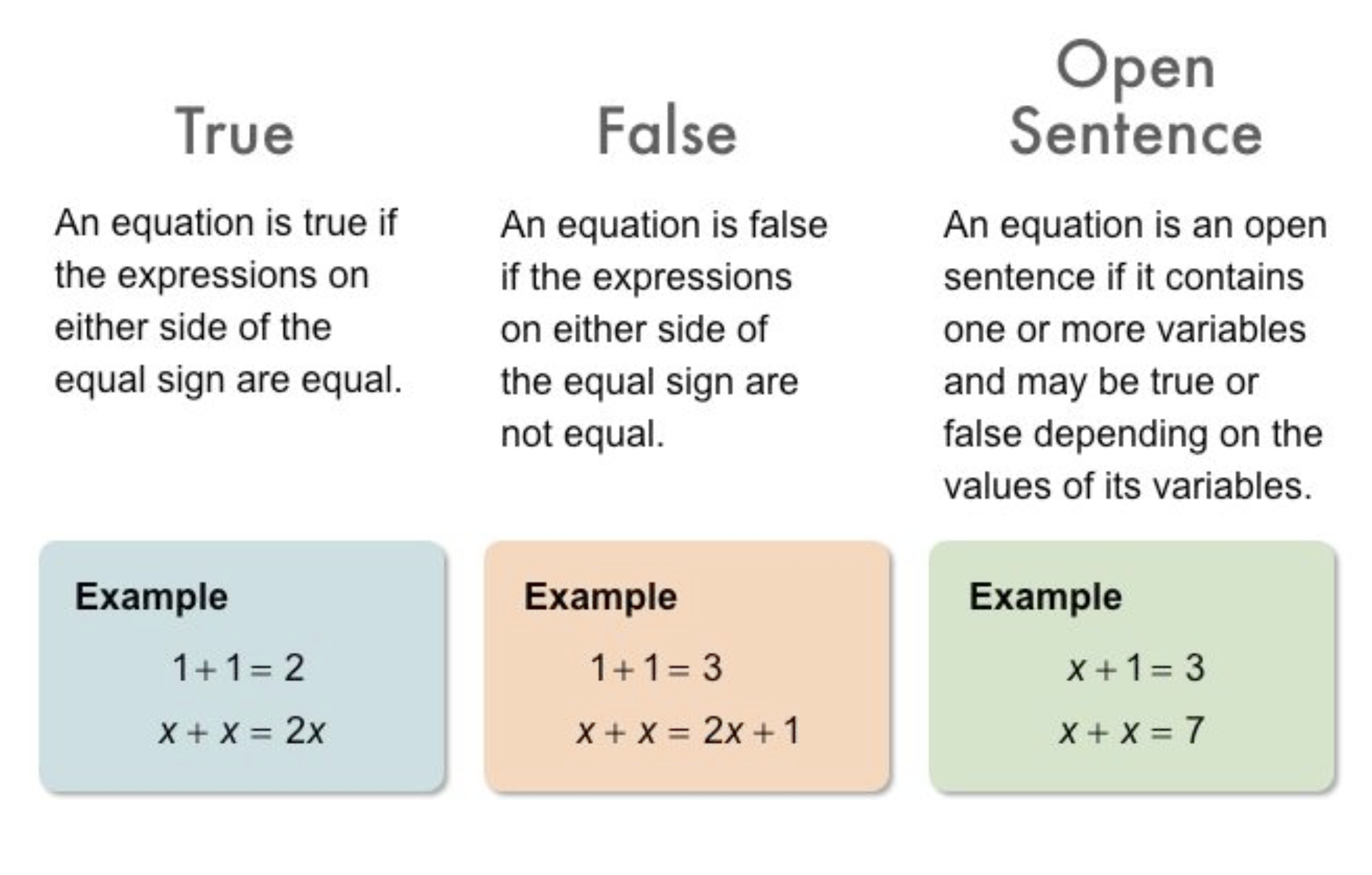2
2
10 pts
Problem 1 Got It?
A
B
C
D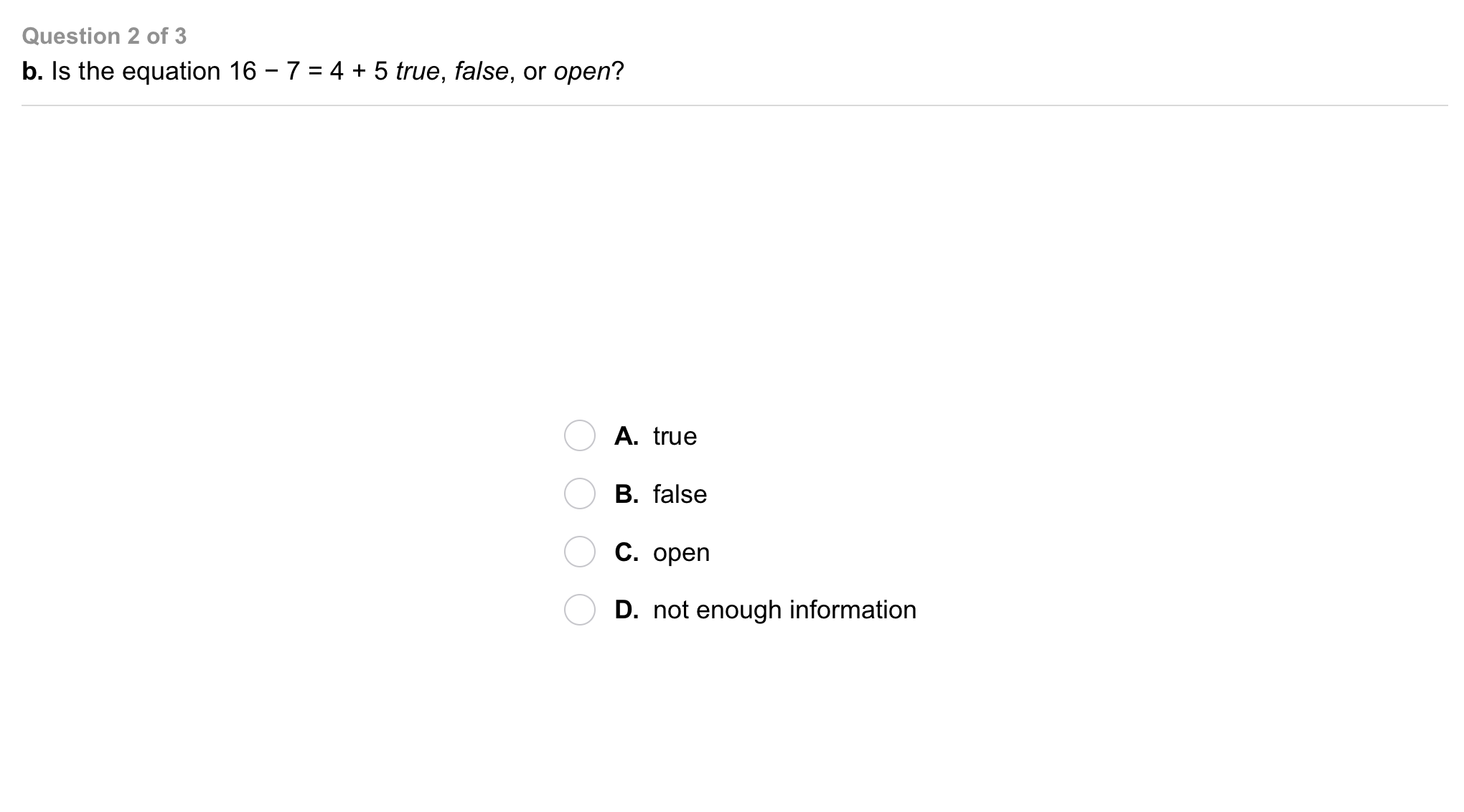3
3
10 pts
Problem 1 Got It?
A
B
C
D4
4
10 pts
Problem 1 Got It?
A
B
C
D5
5
10 pts
Problem 2 Got It?
A
B6
6
10 pts
Problem 3 Got It?
A
B
C
D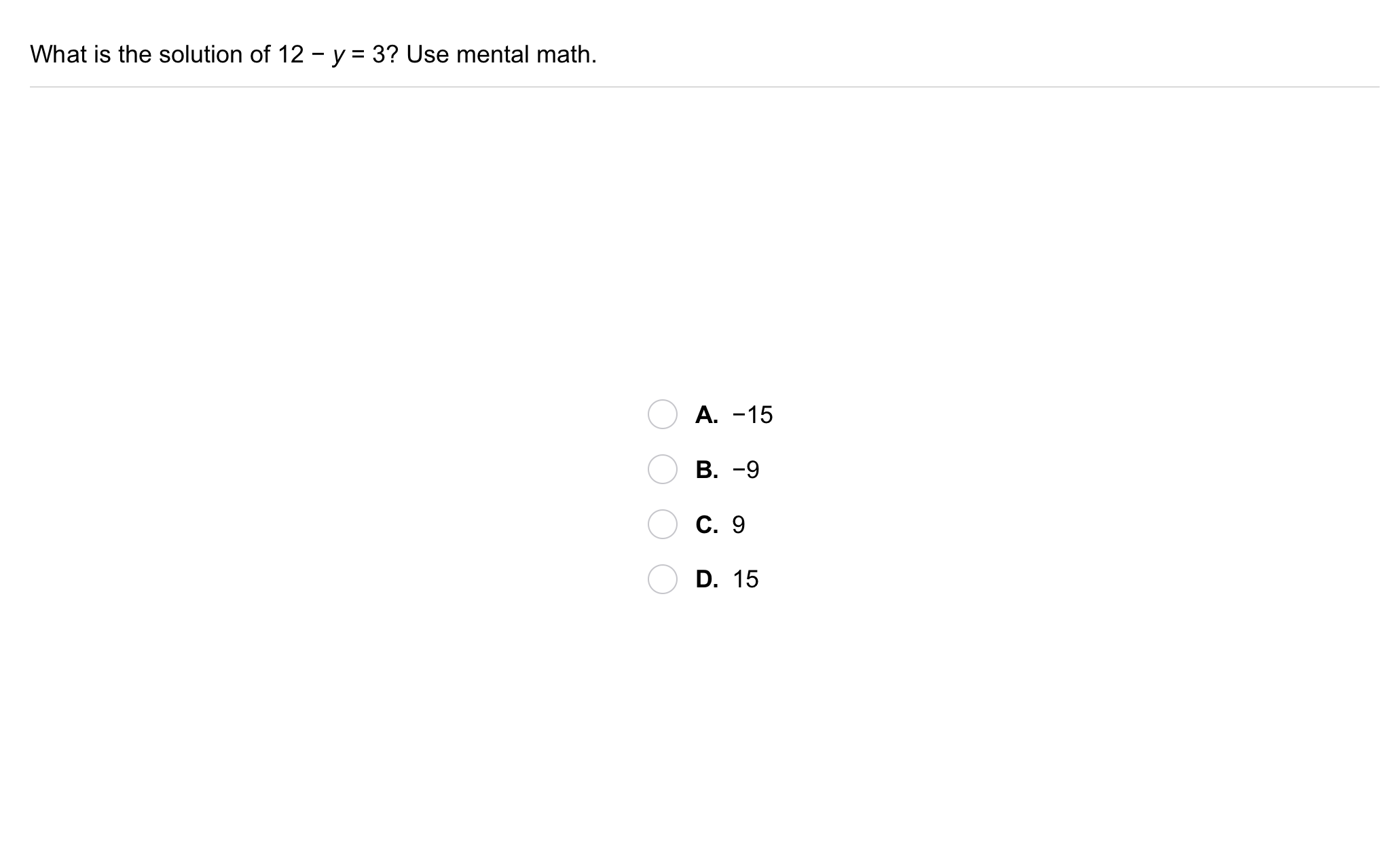7
7
10 pts
Problem 4 Got It?
A
B
C
D
8
50 pts
Problem 5 Got It?9
9
10 pts
Problem 6 Got It?
A
B
C
D10
10
5 pts
A
B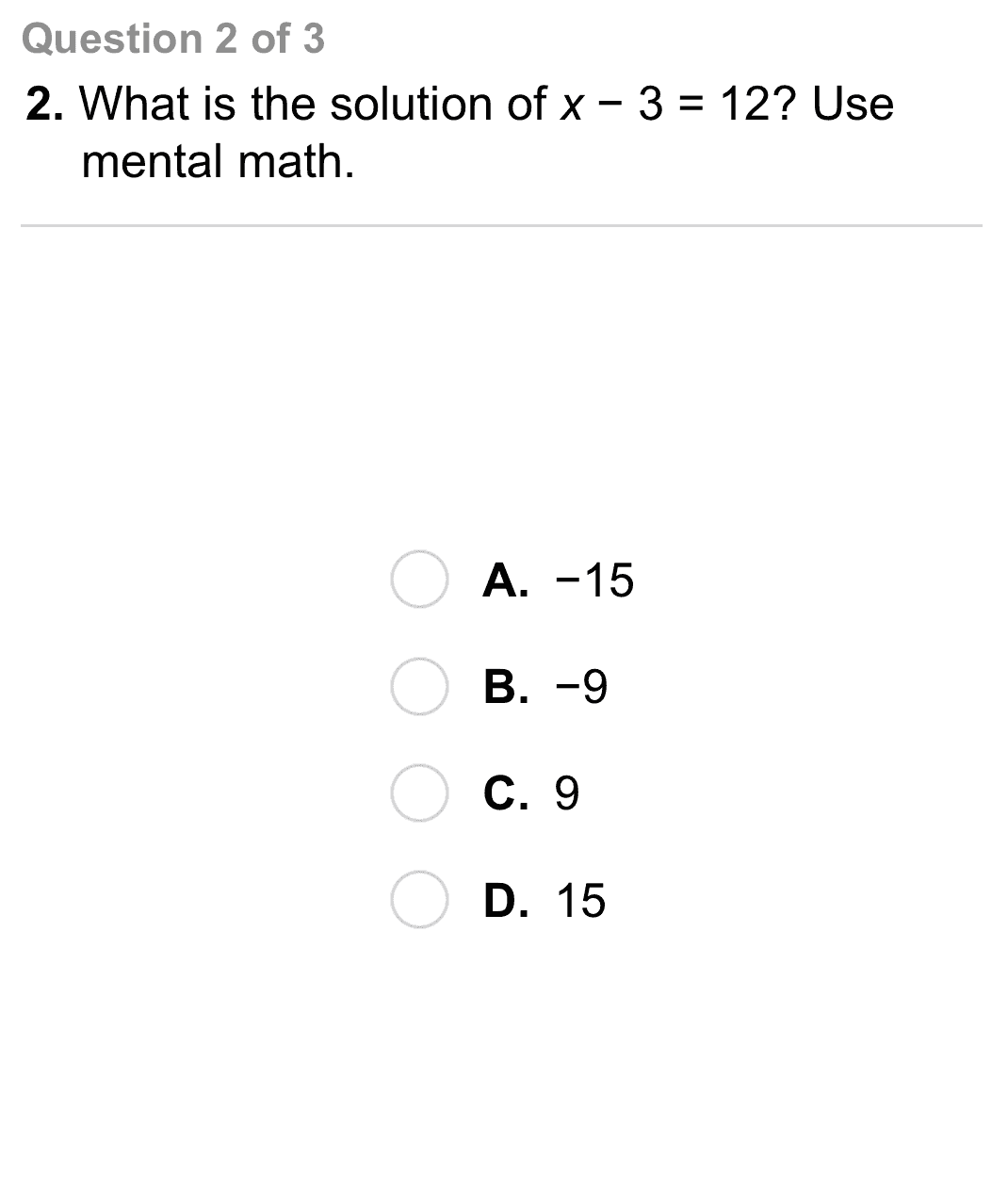11
11
10 pts
A
B
C
D12
12
10 pts
A
B
C
D13
10 pts
Equation Types: Classify each equation on the left as true, false, or open.

• True
• False
• Open
14
10 pts
Open-Ended: Write an open equation using one variable and division.
15
10 pts
Compare and Contrast: Show two different methods to find the solution of the equation x + 4 = 13. Which method do you prefer? Explain.16
10 pts
Review Lesson 1-7: Use the distributive property to simplify the expression.

42y
28 + 2y
28 + 14y
74 + 72y
17
10 pts
Review Lesson 1-7: Use the distributive property to simplify the expression.
Write your response in this format: -13b - 94 (with a space on each side of the - sign between the terms).
18
10 pts
Review Lesson 1-6: Evaluate the expression for m = 4, n = -1, and p = -(1/2).

6
10
8
14
19
10 pts
Review Lesson 1-6: Evaluate the expression for m = 4, n = -1, and p = -(1/2).
Enter only a number.20
10 pts
Vocabulary Review: Identify the pair(s) of opposites. Select all that apply.

21
10 pts
Vocabulary Review: An equation is a mathematical sentence that uses an equal sign (=). Identify the equation(s) below. Select all that apply.

22
10 pts
Vocabulary Review: Use mental math to solve the equation.
Enter only a number.
23
10 pts
Vocabulary Review: Use mental math to solve the equation.
Enter only a number.
24
10 pts
Vocabulary Review: Use mental math to solve the equation.
Enter only a number.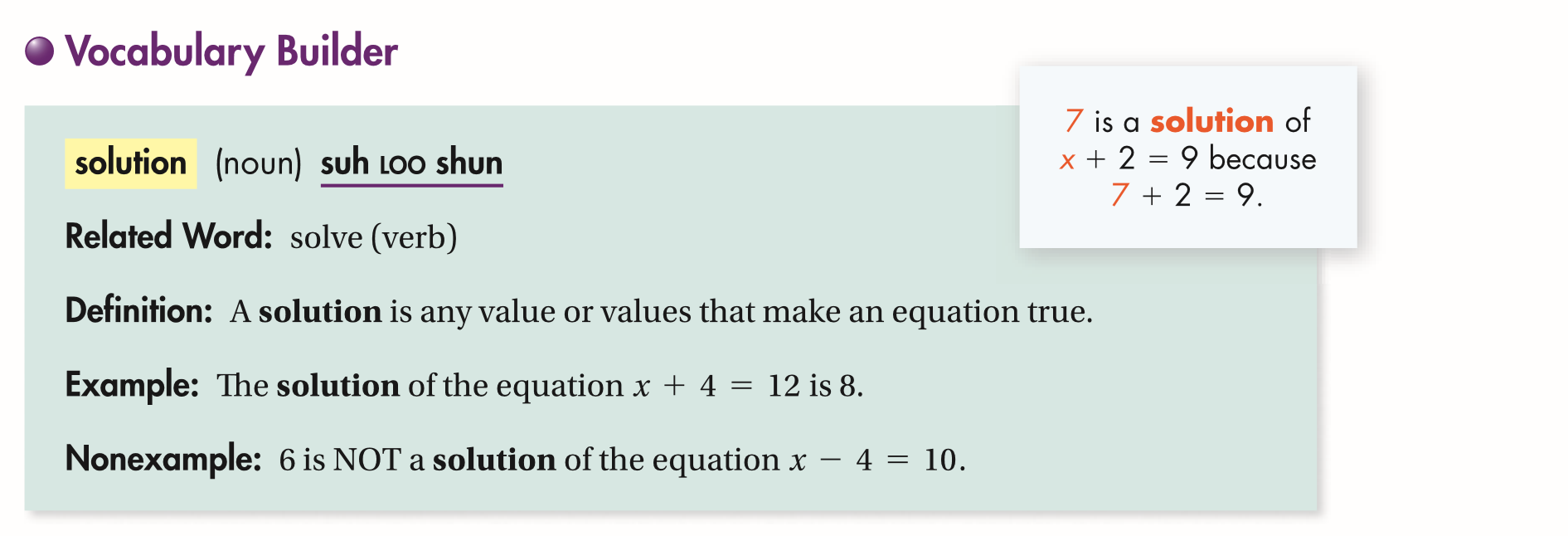25
10 pts
Use Your Vocabulary: Identify the equation for which 24 is NOT the solution.

26
10 pts
Use Your Vocabulary: Identify the equation for which 20 is the solution.

27
10 pts
Use Your Vocabulary: Identify the solution of the equation.

-16
16
2
-228
100 pts
Notes: Take a clear picture or screenshot of your Cornell notes for this lesson. Upload it to the canvas. Zoom and pan as needed.

For a refresher on the Cornell note-taking system, click here.
29
10 pts
Reflection: Math Success
Add to my formatives list

Formative uses cookies to allow us to better understand how the site is used. By continuing to use this site, you consent to the Terms of Service and Privacy Policy.